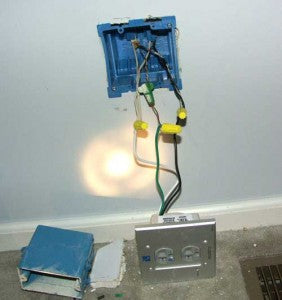# Bits Upon Bits

Prev NextI wish I had something clever to say like “how many bits does it take to screw in a light bulb?” But alas, my simple nerd brain isn’t wired for brilliant jokes. Sorry. What I would say is there seems to be a lot of confusion about bits in digital audio and, while we’re not going to end that confusion just yet, perhaps we can put a dent into it.

Let’s focus today on the PCM version of bits and in a few days we’ll try and tackle how 1-bit systems (DSD) works. For now, let’s look at the counting scheme.

We’ve discussed before that a sample is simply a numeric representation of a voltage level. When the A/D Converter gets a voltage at its input it attempts to assign a number to represent that voltage. Let’s say we have only 8 bits to play with (an 8 bit A/D Converter). If we use binary counting we can count every number from 0 to 256. How? Take a look:This isn’t a great picture but it’s all I could find this morning. The 1’s are an ON state and the 0’s are the off state. The group of numbers is called a digital WORD. If our 8 bit word is all zeros we get the number 0 (00000000). If the word is all zeros except the last bit we get “1” (00000001). If the next to the last bit is a 1, we get “2” (00000010). If the two last bits are 1’s and everything else a zero, they add and we get “3” (00000011). Note the columns go up by a factor of 2 (which is why it’s called binary) 1, 2, 4, 8, 16, 32 etc.

Just stare at the scheme for a moment and you’ll get it. It’s a digital Abacus and this counting scheme’s been around forever.

There’s one main thing to realize looking at this scheme: the number of bits in the word controls how high you can count (the largest possible number).

If we think of the first job of the binary counting scheme, how high we can count, and then relate that to audio, we get the maximum dynamic range and (sort of) signal to noise ratio. Here’s some examples:

• 8 bits = 48dB
• 16 bits = 96dB
• 20 bits = 120dB
• 24 bits = 144dB

That’s pretty easy to get I think – the higher the possible number we can count to the larger the dynamic range – because dynamic range expresses the quietest to the loudest sound and the bigger the number the larger the range.

So here’s one tidbit to take away from this one. As you lose bits, the loss is on the quietest sounds, not the loudest. This could be the opposite, but the way digital audio systems are set up if you record something at 20 bits and then lop off 4 of those bits later on, the loudest signals present are untouched but the quietest signals are just gone – so you would go from 120dB of range (between the loudest to the softest) to 96dB – but the loudest would remain the same.

Lastly, and this is one that people get whacked about, the resolution between steps (each “step” is one of the possible numbers). Let’s go back to our 8-bit example. With 8-bits to play with we have a total of 256 numbers (steps) we can divide the voltage up with. If the number is all the way loud (11111111) then that’s the loudest we can get. But how loud is that? This depends on the voltage you are dividing up in steps. Each step in this system is the identical division. Remember our counting scheme columns were in 2’s? 1, 2, 4, 8, 16, 32 etc. So while the steps are always the same, the size of what we’re dividing can change. We can divide 1 volt up into 256 steps or we can divide 100 volts by the same number. If we use 1 volt vs. 100 volts in our example, the steps size is 10 times larger in the 100 volt example – but the magnitude between each step is identical. I hope that’s not too confusing.

I gotta get to work this morning and I apologize for the brevity of this explanation.

Paul McGowan

Founder & CEO

Paul McGowan

Paul McGowan

Paul McGowan

Paul McGowan

Paul McGowan